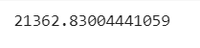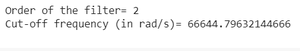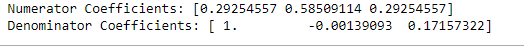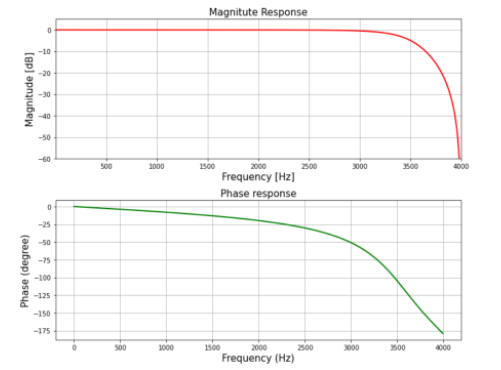Open in App
Not now

# Design IIR Lowpass Butterworth Filter using Bilinear Transformation Method in Scipy- Python

• Last Updated : 16 Dec, 2021

IIR stands for Infinite Impulse Response, It is one of the striking features of many linear-time invariant systems that are distinguished by having an impulse response h(t)/h(n) which does not become zero after some point but instead continues infinitely.

## What is IIR Lowpass Butterworth ?

It basically behaves just like an ordinary digital Lowpass Butterworth Filter with an infinite impulse response.

The specifications are as follows:

• Sampling rate of 8 kHz
• Order of Filter 2
• Cutoff-frequency 3400Hz

We will plot the magnitude & phase response of the filter.

Step-by-step Approach:

Step 1: Importing all the necessary libraries.

## Python3

 `# import required library` `import` `numpy as np` `import` `scipy.signal as signal` `import` `matplotlib.pyplot as plt`

Step 2: Define variables with the given specifications of the filter.

## Python3

 `# Given specification` `N ``=` `2`  `# Order of the filter` `Fs ``=` `8000`  `# Sampling frequency in Hz` `fc ``=` `3400`  `# Cut-off frequency in Hz`   `# Compute Design Sampling parameter` `Td ``=` `1``/``Fs`

Step 3: Computing the cut-off frequency

## Python3

 `# Compute cut-off frequency in radian/sec` `wd ``=` `2``*``np.pi``*``fc` `print``(wd)  ``# Cut-off frequency in radian/sec`

Output:Step 4: Pre-wrapping the analog frequency

## Python3

 `# Prewarp the analog frequency`   `wc ``=` `(``2``/``Td)``*``np.tan(wd``*``Td``/``2``)` `print``(``'Order of the filter='``, N)  ``# Order`   `# Prewarped analog cut-off frequency` `print``(``'Cut-off frequency (in rad/s)='``, wc)`

Output:Step 5: Designing the filter using signal.butter() function and then performing bilinear transformation using signal.bilinear() function

## Python3

 `# Design analog Butterworth filter using signal.butter function`   `b, a ``=` `signal.butter(N, wc, ``'low'``, analog``=``'True'``)` `# Perform bilinear Transformation`   `z, p ``=` `signal.bilinear(b, a, fs``=``Fs)`   `# Print numerator and denomerator coefficients of the filter` `print``(``'Numerator Coefficients:'``, z)` `print``(``'Denominator Coefficients:'``, p)`

Output:Step 6: Computing the frequency response of the filter using signal.freqz() function and plotting the magnitude and phase response

## Python3

 `# Compute frequency response of the filter using signal.freqz function` `wz, hz ``=` `signal.freqz(z, p, ``512``)`   `# Plot filter magnitude and phase responses using subplot.` `# Convert digital frequency wz into analog frequency in Hz` `fig ``=` `plt.figure(figsize``=``(``12``, ``10``))`   `# Calculate Magnitude from hz in dB` `Mag ``=` `20``*``np.log10(``abs``(hz))`   `# Calculate frequency in Hz from wz` `Freq ``=` `wz``*``Fs``/``(``2``*``np.pi)`   `# Plot Magnitude response` `sub1 ``=` `plt.subplot(``2``, ``1``, ``1``)` `sub1.plot(Freq, Mag, ``'r'``, linewidth``=``2``)` `sub1.axis([``1``, Fs``/``2``, ``-``60``, ``5``])` `sub1.set_title(``'Magnitude Response'``, fontsize``=``15``)` `sub1.set_xlabel(``'Frequency [Hz]'``, fontsize``=``15``)` `sub1.set_ylabel(``'Magnitude [dB]'``, fontsize``=``15``)` `sub1.grid()`     `# Plot phase angle` `sub2 ``=` `plt.subplot(``2``, ``1``, ``2``)`   `# Calculate phase angle in degree from hz` `Phase ``=` `np.unwrap(np.angle(hz))``*``180``/``np.pi` `sub2.plot(Freq, Phase, ``'g'``, linewidth``=``2``)` `sub2.set_ylabel(``'Phase (degree)'``, fontsize``=``15``)` `sub2.set_xlabel(r``'Frequency (Hz)'``, fontsize``=``15``)` `sub2.set_title(r``'Phase response'``, fontsize``=``15``)` `sub2.grid()`   `plt.subplots_adjust(hspace``=``0.5``)` `fig.tight_layout()` `plt.show()`

Output:Below is the implementation:

## Python3

 `# import required library` `import` `numpy as np` `import` `scipy.signal as signal` `import` `matplotlib.pyplot as plt`   `# Given specification` `N ``=` `2`  `# Order of the filter` `Fs ``=` `8000`  `# Sampling frequency in Hz` `fc ``=` `3400`  `# Cut-off frequency in Hz`   `# Compute Design Sampling parameter` `Td ``=` `1``/``Fs`   `# Compute cut-off frequency in radian/sec` `wd ``=` `2``*``np.pi``*``fc` `print``(wd)  ``# Cut-off frequency in radian/sec`   `# Prewarp the analog frequency` `wc ``=` `(``2``/``Td)``*``np.tan(wd``*``Td``/``2``)` `print``(``'Order of the filter='``, N)  ``# Order`   `# Prewarped analog cut-off frequency` `print``(``'Cut-off frequency (in rad/s)='``, wc)`   `# Design analog Butterworth filter using signal.butter function` `b, a ``=` `signal.butter(N, wc, ``'low'``, analog``=``'True'``)`   `# Perform bilinear Transformation` `z, p ``=` `signal.bilinear(b, a, fs``=``Fs)`   `# Print numerator and denomerator coefficients of the filter` `print``(``'Numerator Coefficients:'``, z)` `print``(``'Denominator Coefficients:'``, p)`   `# Compute frequency response of the filter using signal.freqz function` `wz, hz ``=` `signal.freqz(z, p, ``512``)`   `# Plot filter magnitude and phase responses using subplot.` `#Convert digital frequency wz into analog frequency in Hz` `fig ``=` `plt.figure(figsize``=``(``10``, ``8``))`   `# Calculate Magnitude from hz in dB` `Mag ``=` `20``*``np.log10(``abs``(hz))`   `# Calculate frequency in Hz from wz` `Freq ``=` `wz``*``Fs``/``(``2``*``np.pi)`   `# Plot Magnitude response` `sub1 ``=` `plt.subplot(``2``, ``1``, ``1``)` `sub1.plot(Freq, Mag, ``'r'``, linewidth``=``2``)` `sub1.axis([``1``, Fs``/``2``, ``-``60``, ``5``])` `sub1.set_title(``'Magnitude Response'``, fontsize``=``15``)` `sub1.set_xlabel(``'Frequency [Hz]'``, fontsize``=``15``)` `sub1.set_ylabel(``'Magnitude [dB]'``, fontsize``=``15``)` `sub1.grid()`   `# Plot phase angle` `sub2 ``=` `plt.subplot(``2``, ``1``, ``2``)`   `# Calculate phase angle in degree from hz` `Phase ``=` `np.unwrap(np.angle(hz))``*``180``/``np.pi` `sub2.plot(Freq, Phase, ``'g'``, linewidth``=``2``)` `sub2.set_ylabel(``'Phase (degree)'``, fontsize``=``15``)` `sub2.set_xlabel(r``'Frequency (Hz)'``, fontsize``=``15``)` `sub2.set_title(r``'Phase response'``, fontsize``=``15``)` `sub2.grid()`   `plt.subplots_adjust(hspace``=``0.5``)` `fig.tight_layout()` `plt.show()`

Output:My Personal Notes arrow_drop_up
Related Articles